#### Sampling rate

For the first problem, time is discretized into steps, in which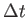is the step size or sampling rate. For example,might be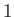ms, in which case the state can be calculated for times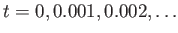, in terms of seconds. This can be considered as a kind of frame rate for the physics engine. Eachcorresponds to the production of a new frame.

As mentioned in Section 7.4, the VWG should synchronize the production of virtual world frames with rendering processes so that the world is not caught in an intermediate state with some variables updated to the new time and others stuck at the previous time. This is a kind of tearing in the virtual world. This does not, however, imply that the frame rates are the same between renderers and the physics engine. Typically, the frame rate for the physics engine is much higher to improve numerical accuracy.

Using the sampling rate, (8.20) is approximated as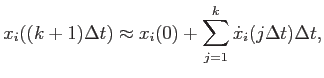(8.21)

for each state variable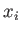.

It is simpler to view (8.21) one step at a time. Let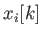denote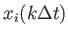, which is the state at time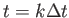. The following is an update law that expresses the new state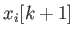in terms of the old state: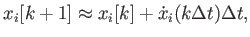(8.22)

which starts with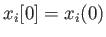.

Steven M LaValle 2020-11-11